## Physically Interpretable Machine Learning for nuclear masses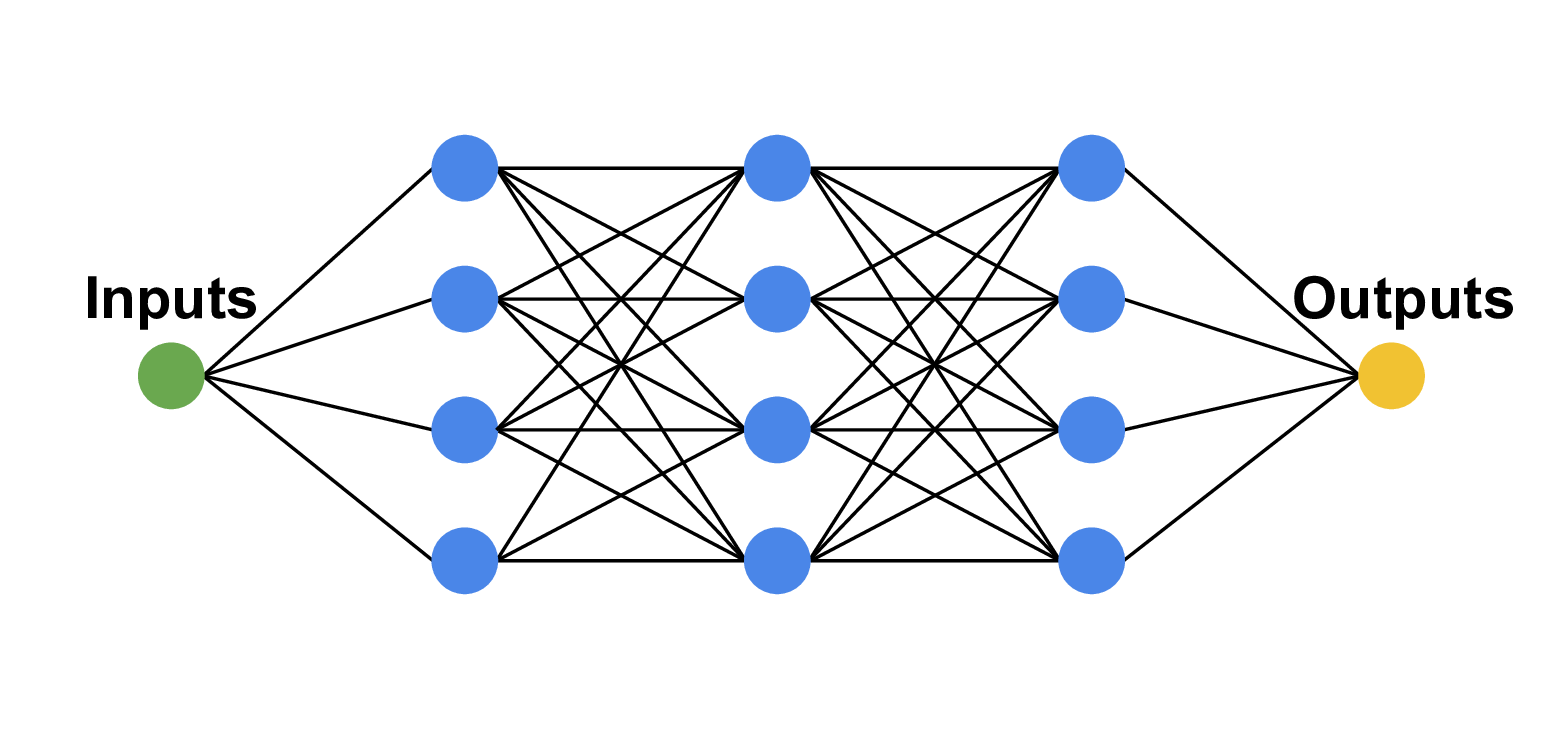LA-UR-22-22701

### Matthew Mumpower

INT ML Nuclear Physics Workshop

Thursday March 31$^{st}$ 2022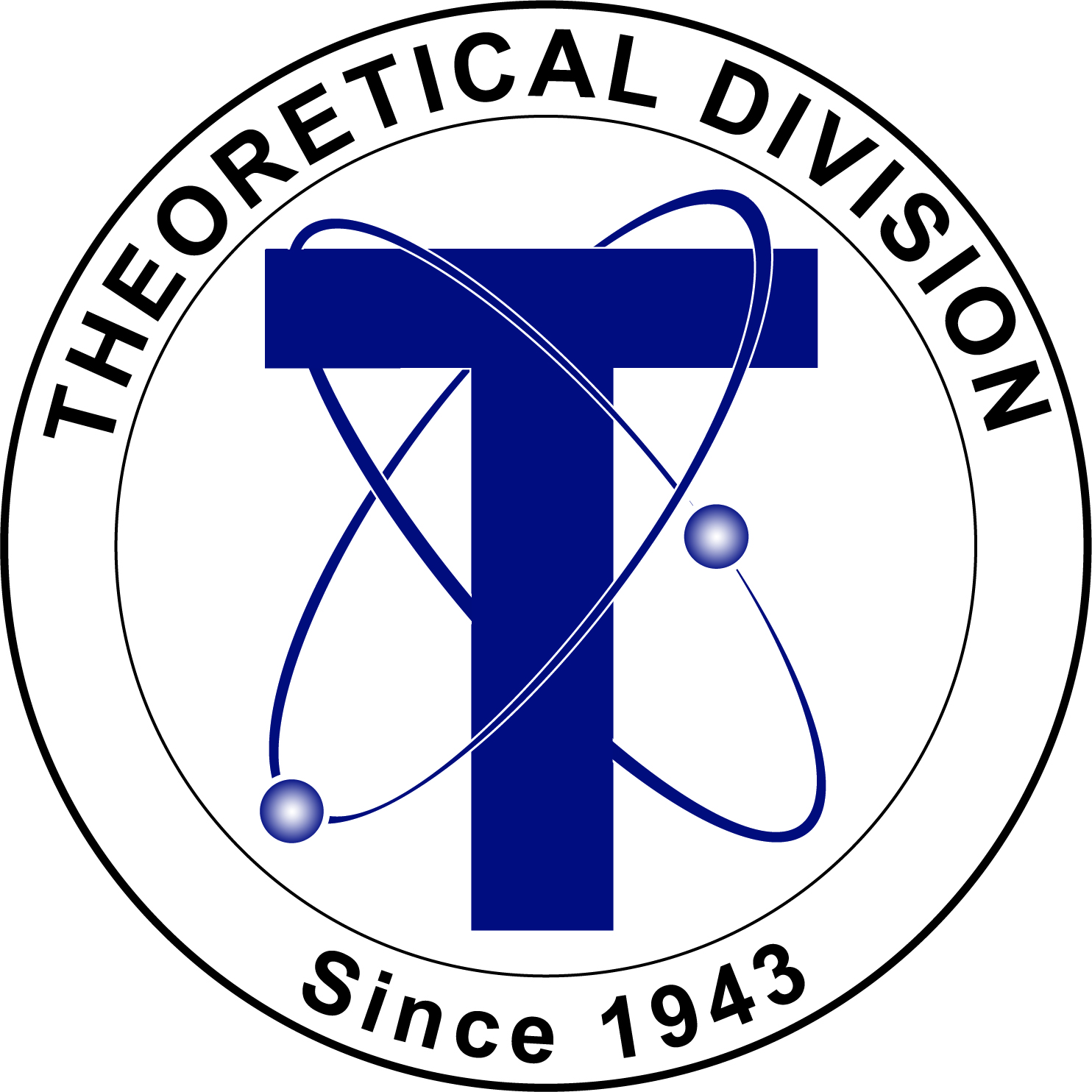Theoretical DivisionLos Alamos National Laboratory Caveat

The submitted materials have been authored by an employee or employees of Triad National Security, LLC (Triad) under contract with the U.S. Department of Energy/National Nuclear Security Administration (DOE/NNSA).

Accordingly, the U.S. Government retains an irrevocable, nonexclusive, royaltyfree license to publish, translate, reproduce, use, or dispose of the published form of the work and to authorize others to do the same for U.S. Government purposes.

## Nuclear Physics is Ubiquitous in modern applications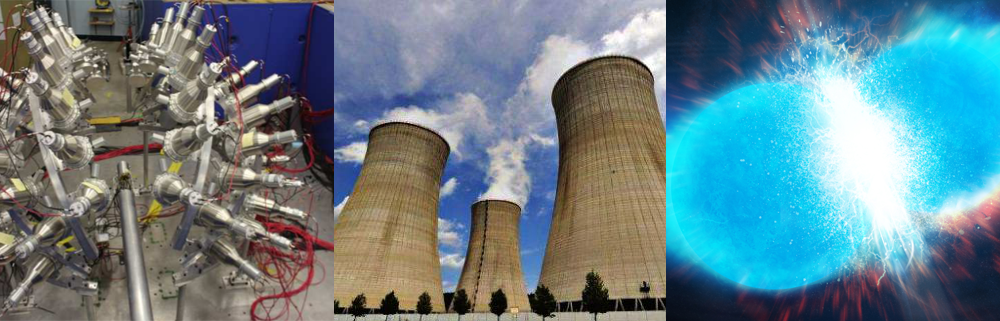Example: fission yields are needed for a variety of applications and cutting-edge science

Industrial applications: simulation of reactors, fuel cycles, waste management

Experiments: backgrounds, isotope production with radioactive ion beams (fragmentation)

Science applications: nucleosynthesis, light curve observations

Other Applications: national security, nonproliferation, nuclear forensics

Figure: Chi-Nu detector (left), Reactor cooling towers (middle), Neutron star merger (right)

## Nuclear astrophysics requires nuclear theory

Even in the era of FRIB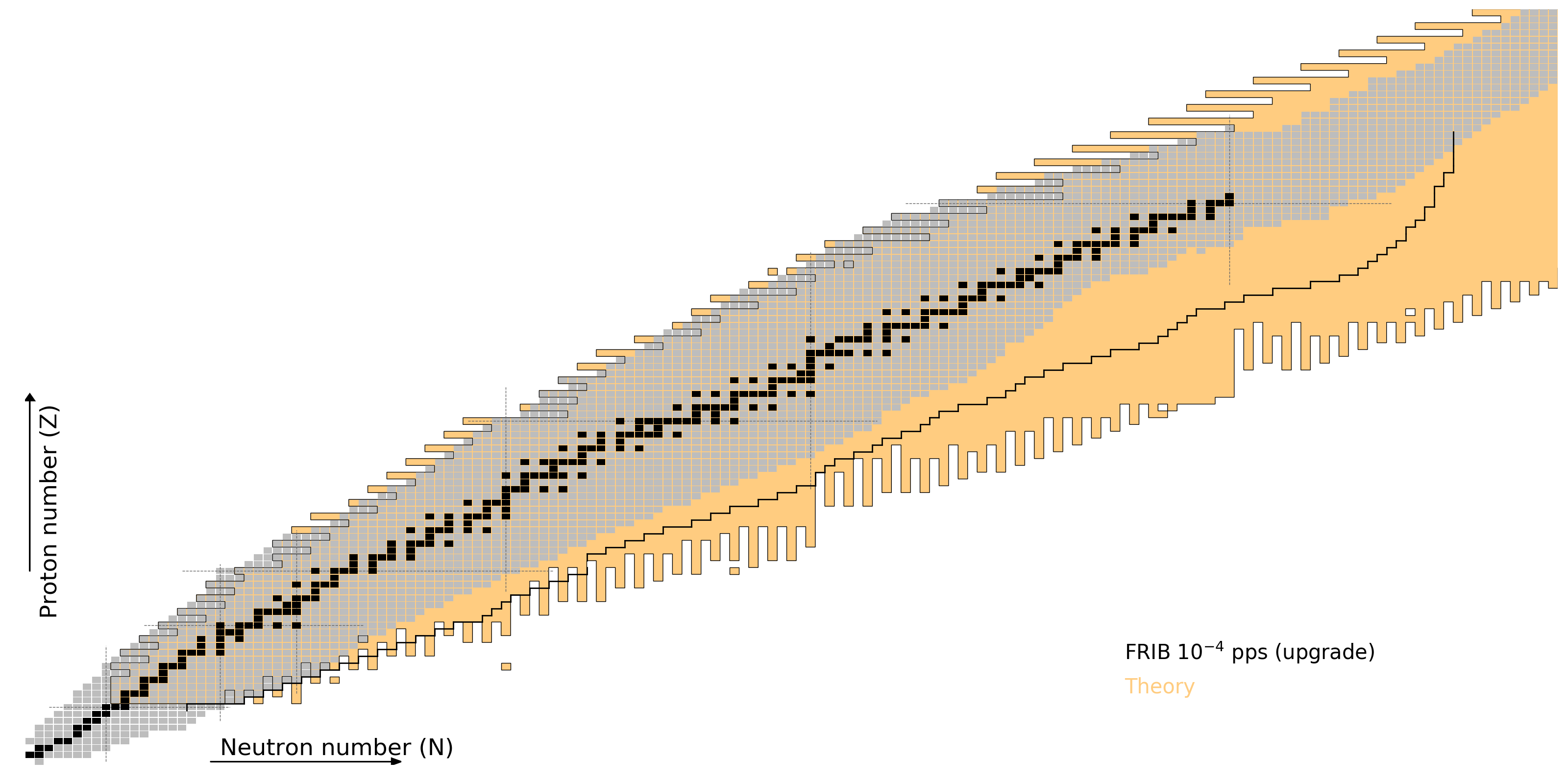Figure by Mumpower

## Nuclear physics from a forward problem perspective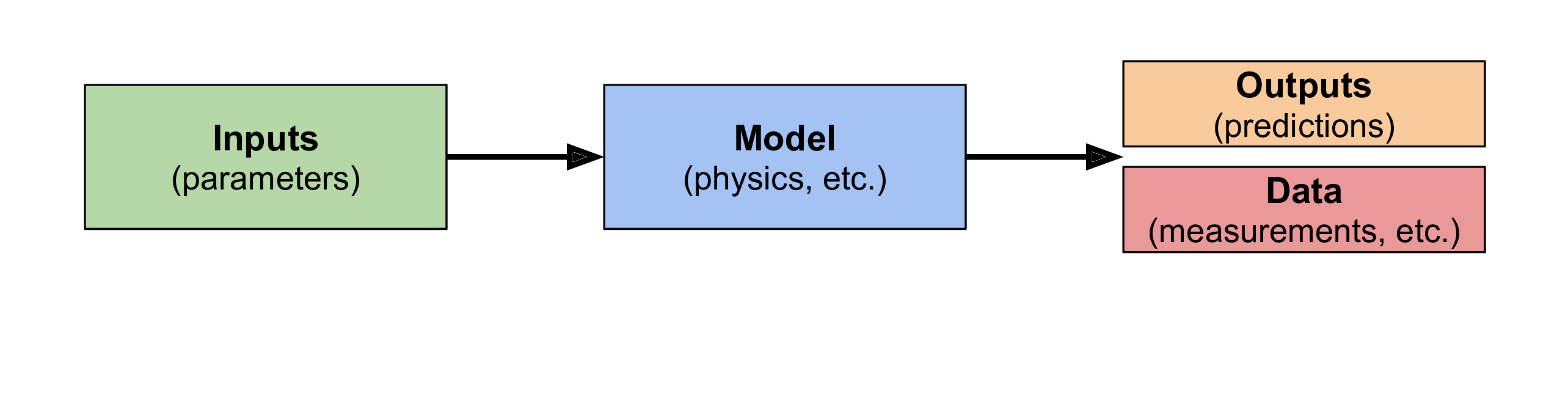When we model nuclear properties we think of it as a 'forward' problem

We start with our model and parameters and try to match measurements / observations

This is an extremely successful approach and is generally how we use theory models

Mathematically: $f(\vec{\boldsymbol{x}}) = \vec{\boldsymbol{y}}$

Where $f$ is our model, $\vec{\boldsymbol{x}}$ are the parameters for the model and $\vec{\boldsymbol{y}}$ are the predictions

Figure by Mumpower

## Forward problem... problems...There can be many challenges with this approach

For instance: model computationally expensive or how do we update our model if we don't match data?

This can be challenging

We don't always know what modifications are required or the physics could be very hard if not impossible to model given current computational limitations (e.g. many-body problem)

Treating this as an 'inverse problem' provides an alternative approach...

Figure by Mumpower

## Nuclear physics from an inverse problem perspective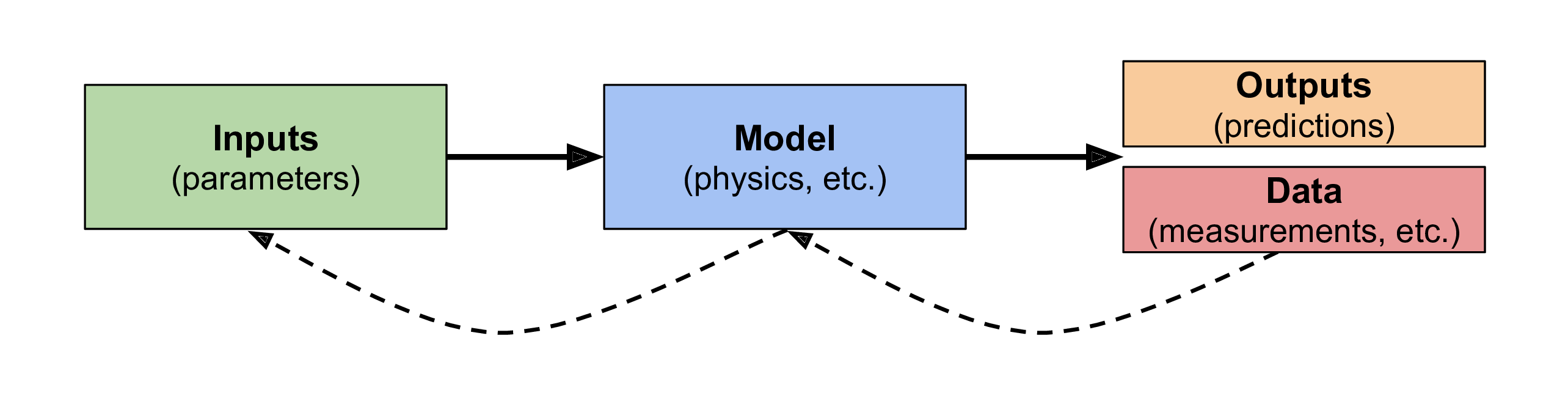We can then try to figure out what models may match relevant observations

This approach allows us to vary and optimize the model!

Mathematically, still: $f(\vec{\boldsymbol{x}}) = \vec{\boldsymbol{y}}$; but we want to now find $f$; fixing $\vec{\boldsymbol{x}}$

Machine learning and artificial intelligence algorithms are ideal for this class of problems...

Figure by Mumpower

## An example of how this works (from my life)

My son Zachary has been very keen on learning his letters from an early age

Letters are well known (this represents the data we want to match to)

But the model (being able to draw - eventually letters) must be developed, practiced and finally optimized

This is a complex, iterative process - it takes times to find '$f$'!

## An example of how this works (from my life)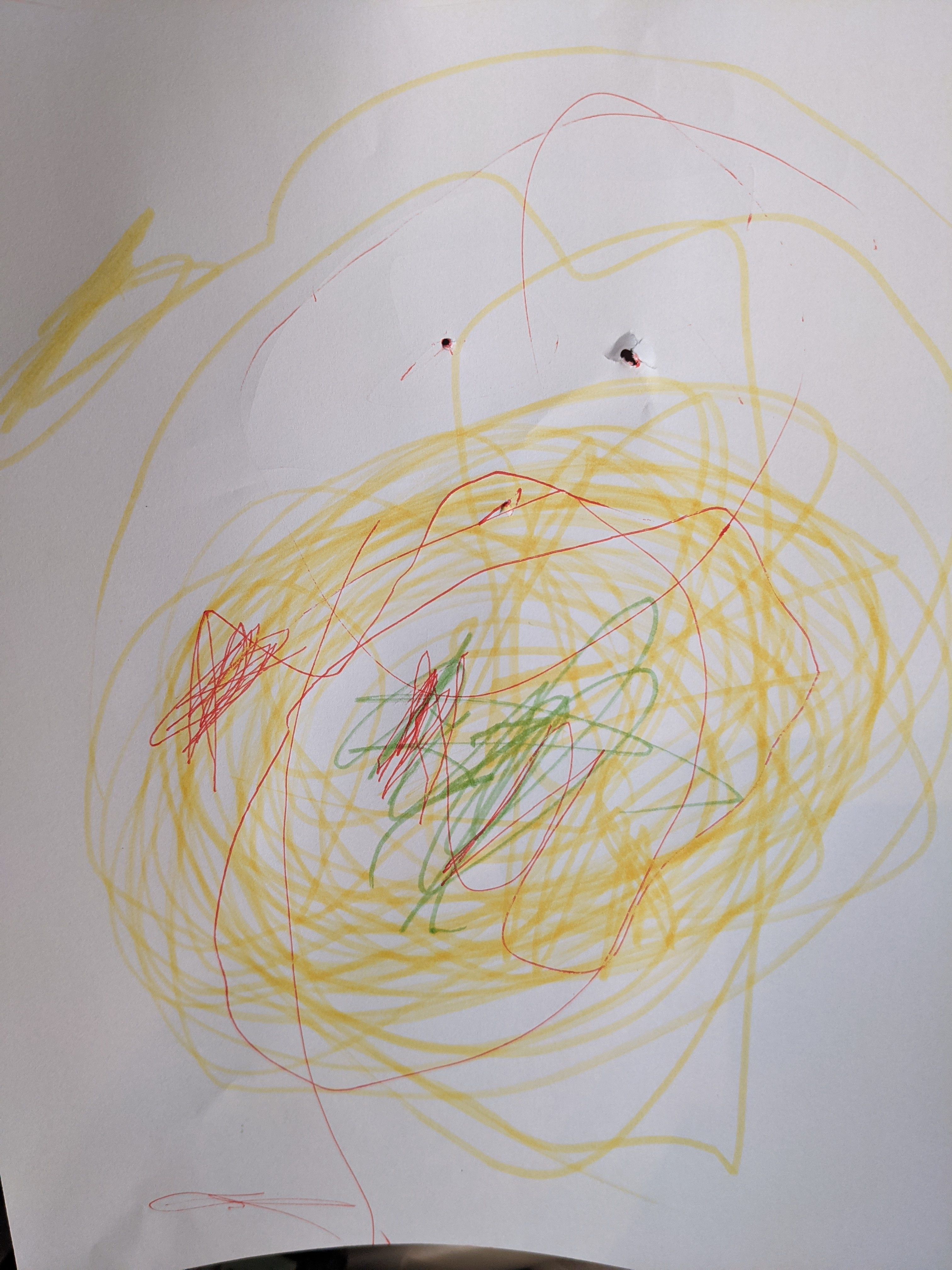Example from 1 years old; random drawing episode; time taken: 10 minutes

Drawing by Z. R. Mumpower

## An example of how this works (from my life)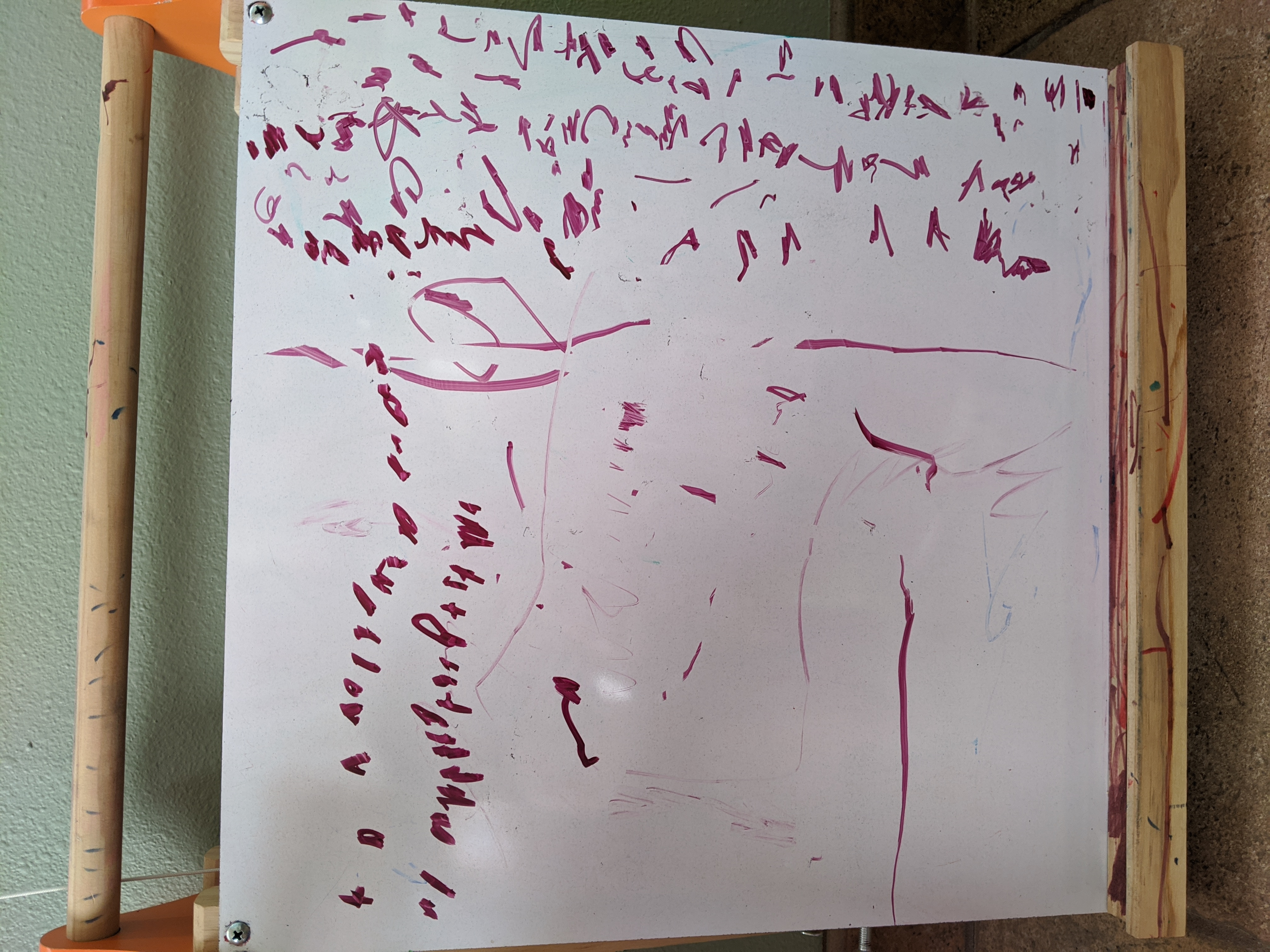Example from 2.5 years old; first ever attempt to draw letters / alphabet; time taken: 2 hours!

Drawing by Z. R. Mumpower

## An example of how this works (from my life)Example from 3.5 years old; drawing scenery; time taken: 10 minutes

Drawing by Z. R. Mumpower

## An example of how this works (from my life)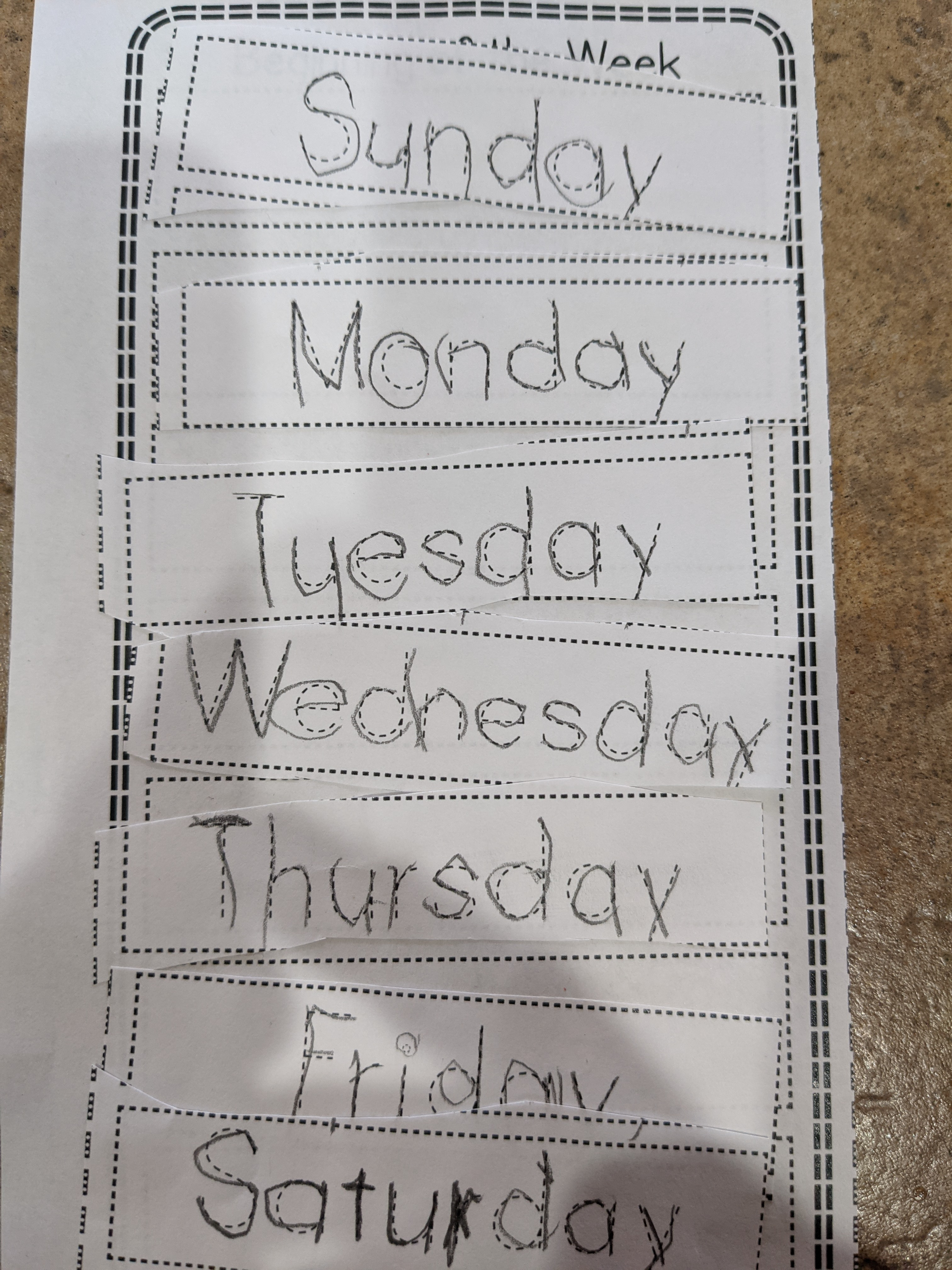Example from 4 years old; tracing the days of the week; time taken: 30 minutes

Drawing by Z. R. Mumpower

## Let's apply this idea to a basic property...

A relatively easy choice is a scalar property; how about atomic binding energies (masses)?

Recall: $f(\vec{\boldsymbol{x}}) = \vec{\boldsymbol{y}}$

$f$ will be a neural network (a model that can change)

$\vec{\boldsymbol{y}_{obs}}$ are the observations (e.g. Atomic Mass Evaluation) we want to match $\vec{\boldsymbol{y}}$ with

But what about $\vec{\boldsymbol{x}}$?

Why not a physically motivated feature space!?

proton number ($Z$), neutron number, ($N$), nucleon number ($A$), etc.

Lovell, Mohan, Sprouse & Mumpower arXiv:2201.00676 (2022)

## Network feature spaces ($\vec{\boldsymbol{x}}$)We use a variety of possible physically motivated features as inputs into the model

Lovell, Mohan, Sprouse & Mumpower arXiv:2201.00676 (2022)

## Mixture Density Network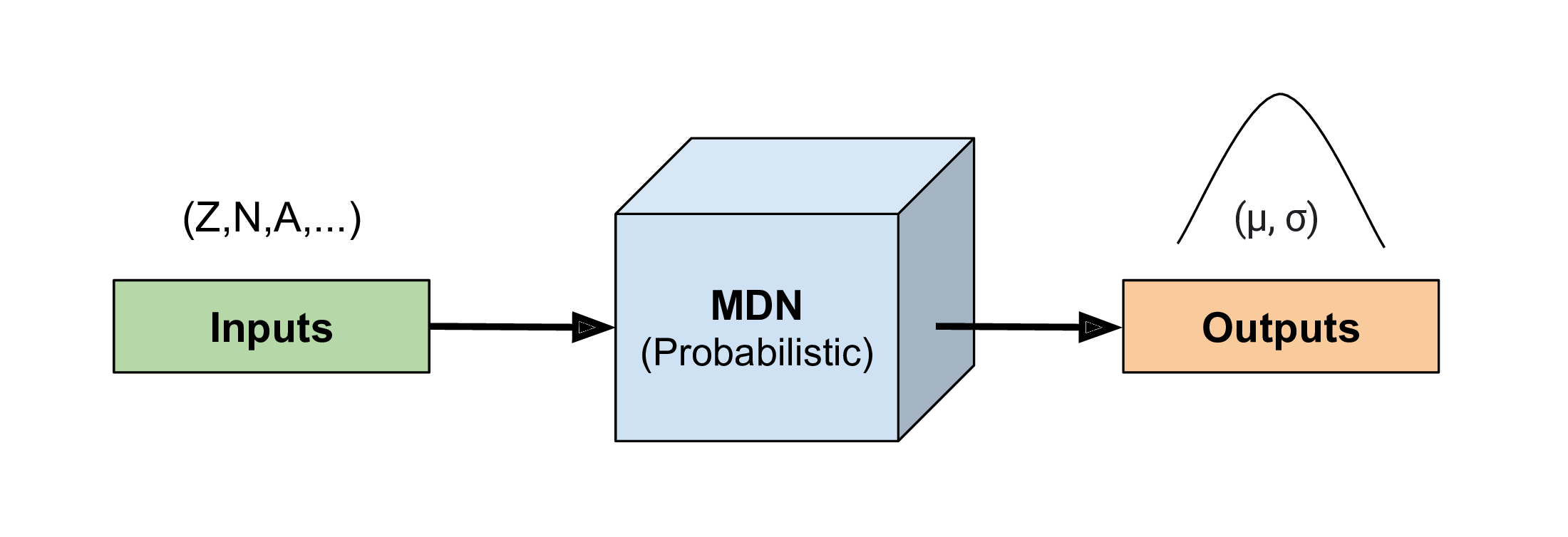We take a Bayesian approach: our network inputs are sampled based on nuclear data uncertainties

Our network outputs are therefore statistical

We can represent outputs by a collection of Gaussians (for masses we only need 1)

Our network also provides a well-quanitfied estimate of uncertainty (fully propagated through the model)

Network topology: fully connected $\sim$4-8 layers; 8 nodes; regularization via weight-decay

Last layer converts output to Gaussian ad-mixture

Bishop (1994 & 2006) • Lovell, Mohan, Sprouse & Mumpower arXiv:2201.00676 (2022)

## Results: modeling atomic masses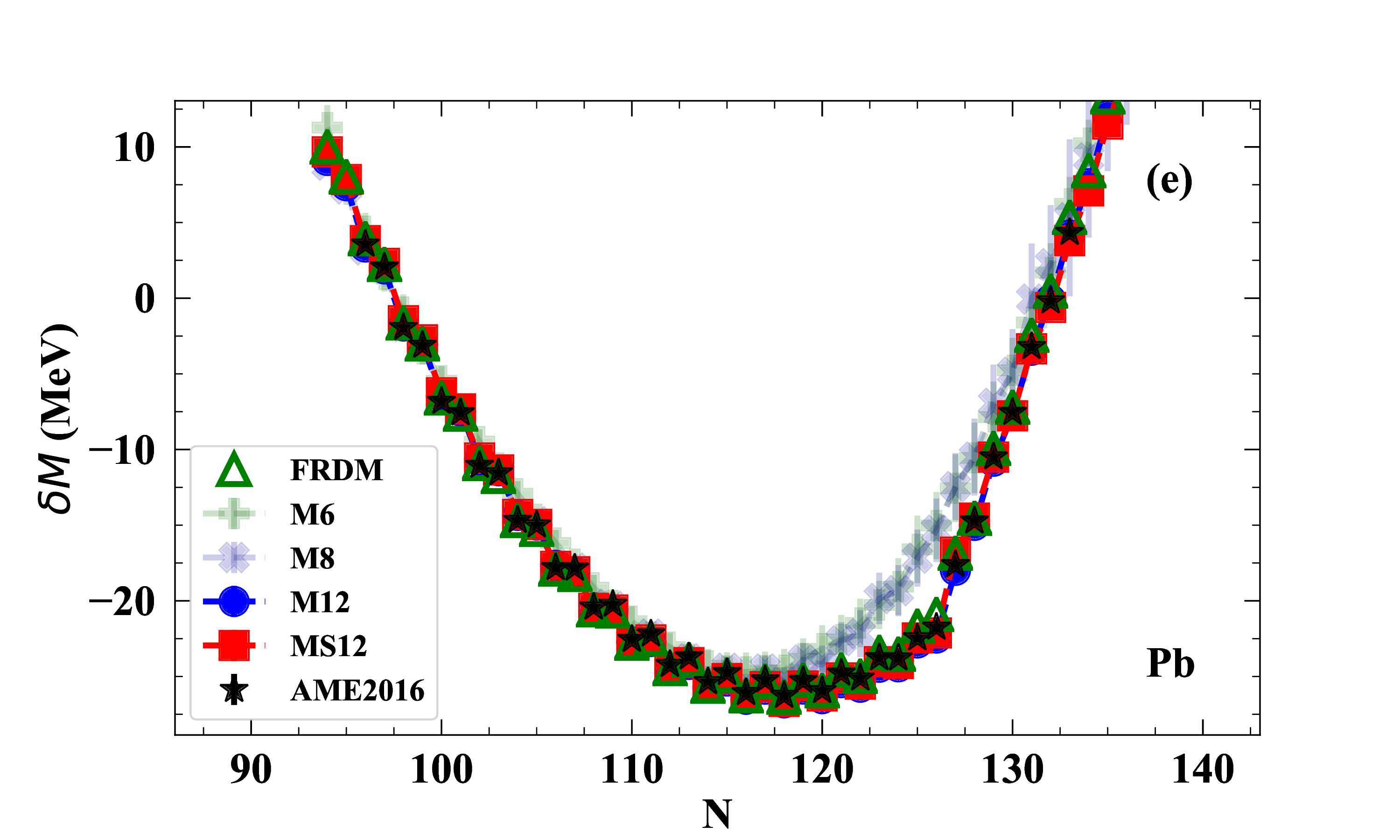Here we show results along the lead (Pb) [$Z=82$] isotopic chain

Data from the Atomic Mass Evaluation (AME2016) in black stars

Increasing sophistication of the model feature space indicated by M##

Lovell, Mohan, Sprouse & Mumpower arXiv:2201.00676 (2022)

## Results: modeling atomic masses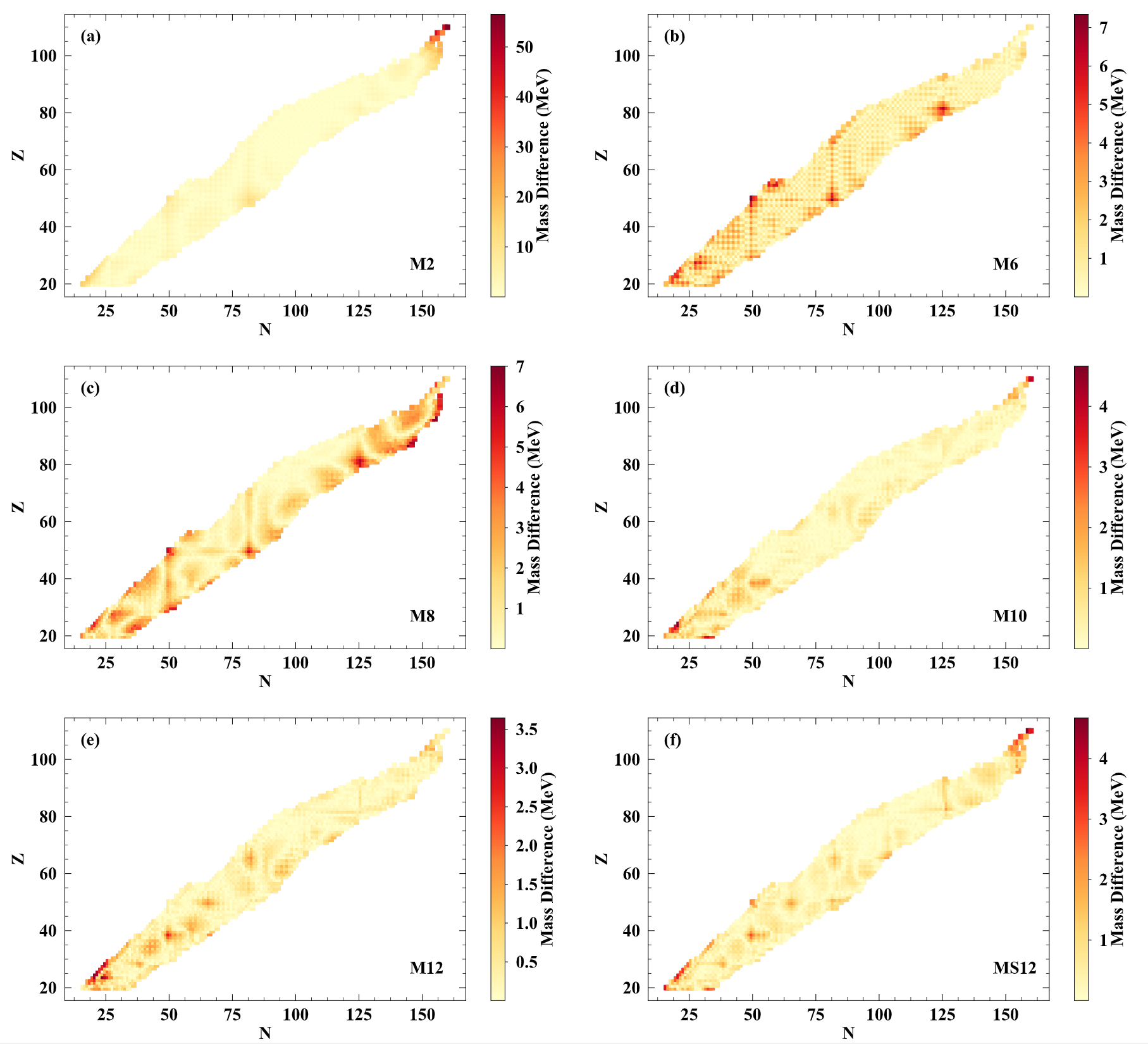Results across the chart of nuclides with increasing feature space complexity

Note the decrease in the maximum value of the scale

Lovell, Mohan, Sprouse & Mumpower arXiv:2201.00676 (2022)

## Results: modeling atomic masses

A summary of results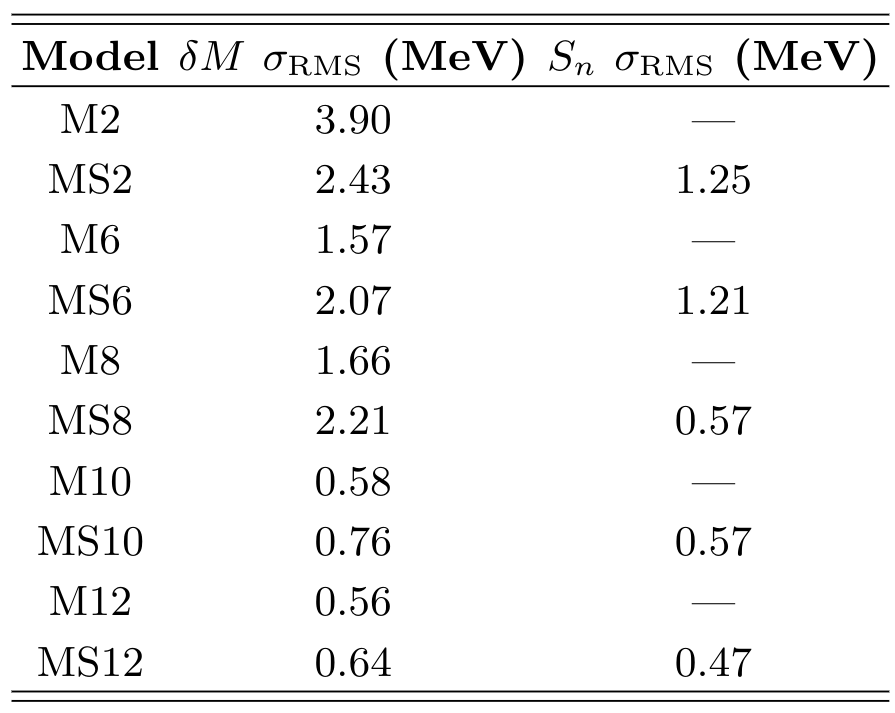Some lessons learned:

More physics added to the feature space → the better our description of atomic masses

Attempting to fit and predict other mass related quantities (e.g. separation energies) does impact our results

Although marginal compared to choice of feature space

Lovell, Mohan, Sprouse & Mumpower arXiv:2201.00676 (2022)

## Training on mass data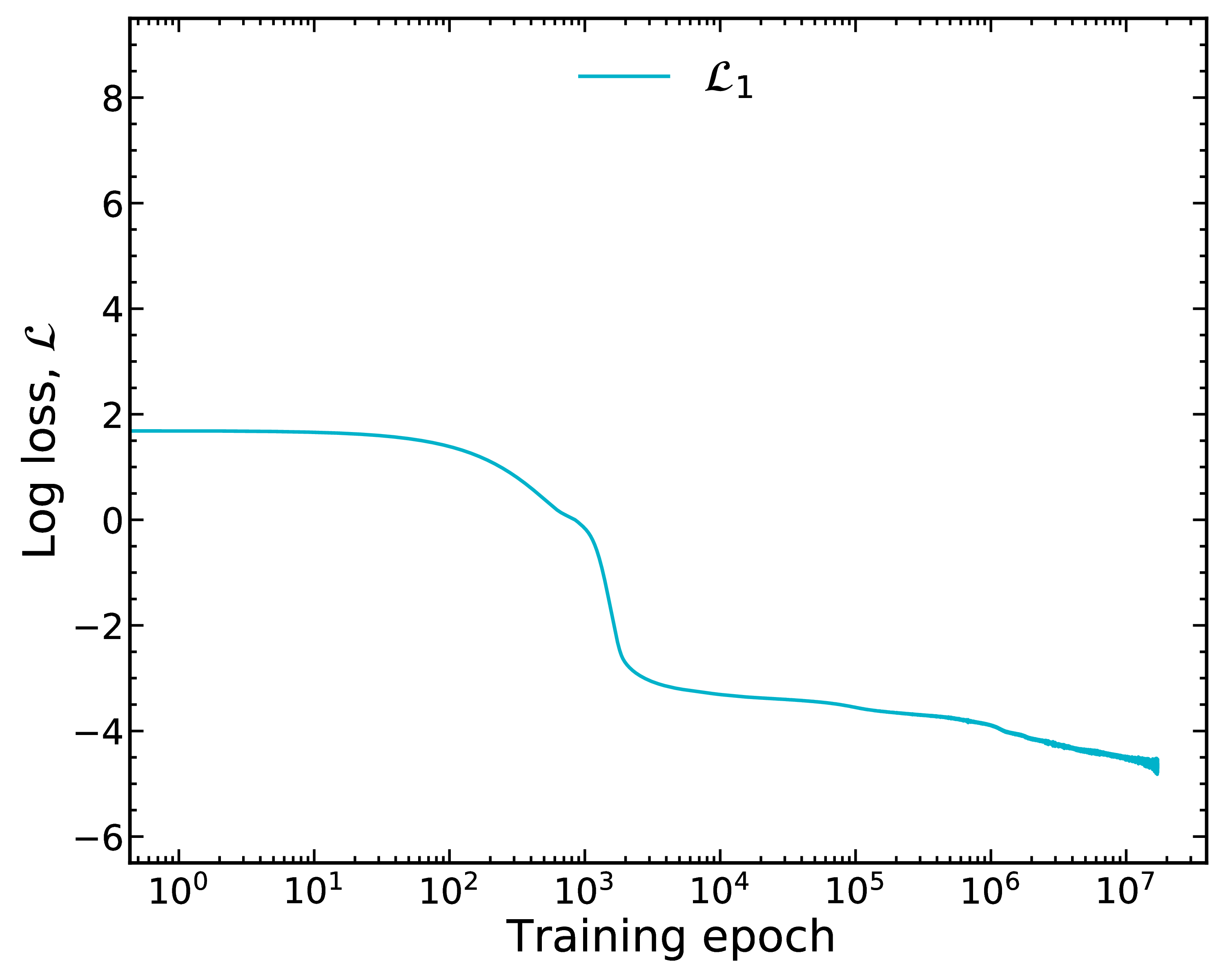We train on mass data using a log-loss, $\mathcal{L}_1$

Training takes a considerably long time

Mumpower, Sprouse, Lovell, & Mohan arXiv:2203.10594 (2022)

## Introduce physical constraint via loss function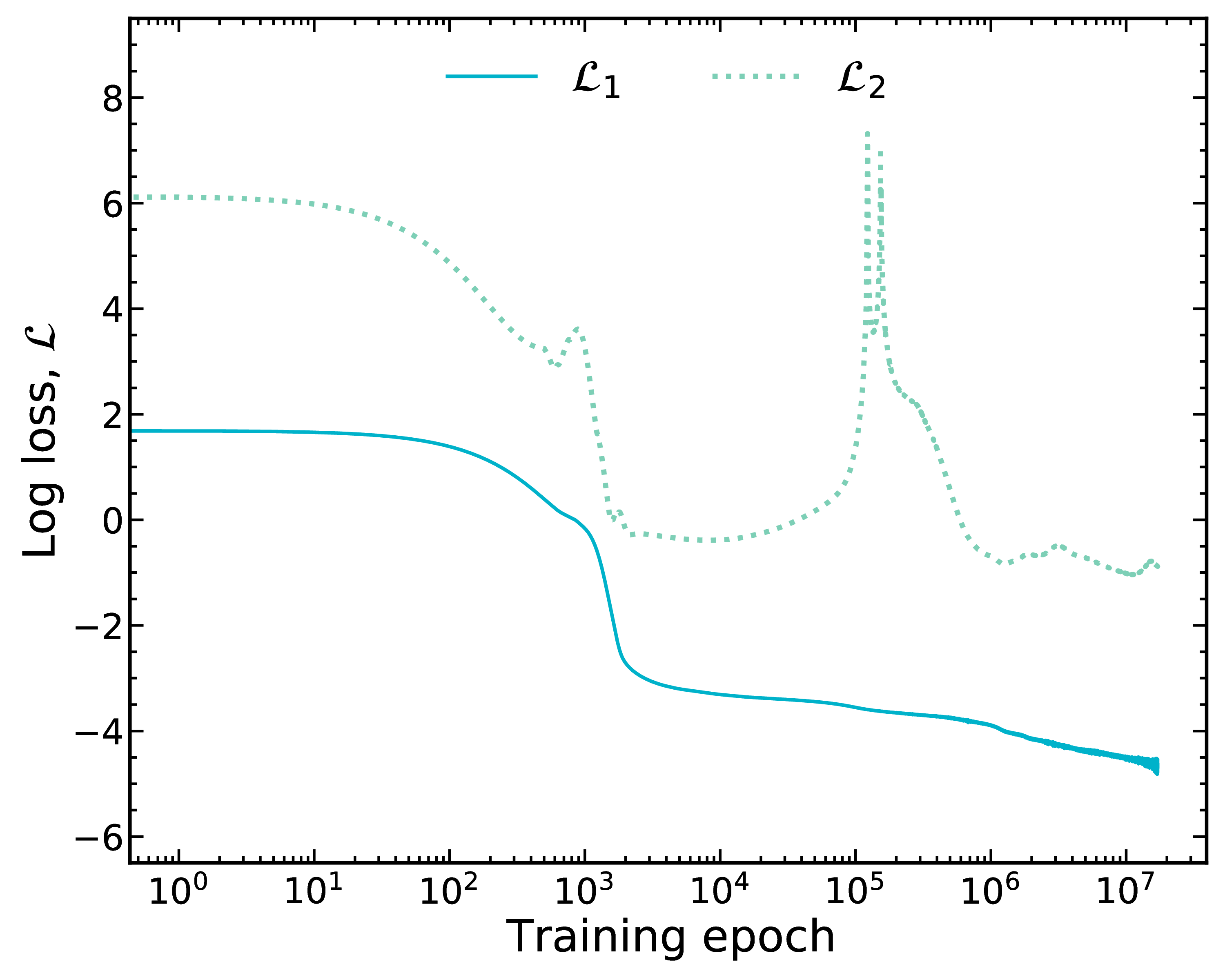Now we optimize data, $\mathcal{L}_1$, and introduce a second (physical) constraint, $\mathcal{L}_2$

We have tried many physics-based losses, this particular one (Garvey-Kelson relations) performs well

Mumpower, Sprouse, Lovell, & Mohan arXiv:2203.10594 (2022)

## Total loss: sum the two losses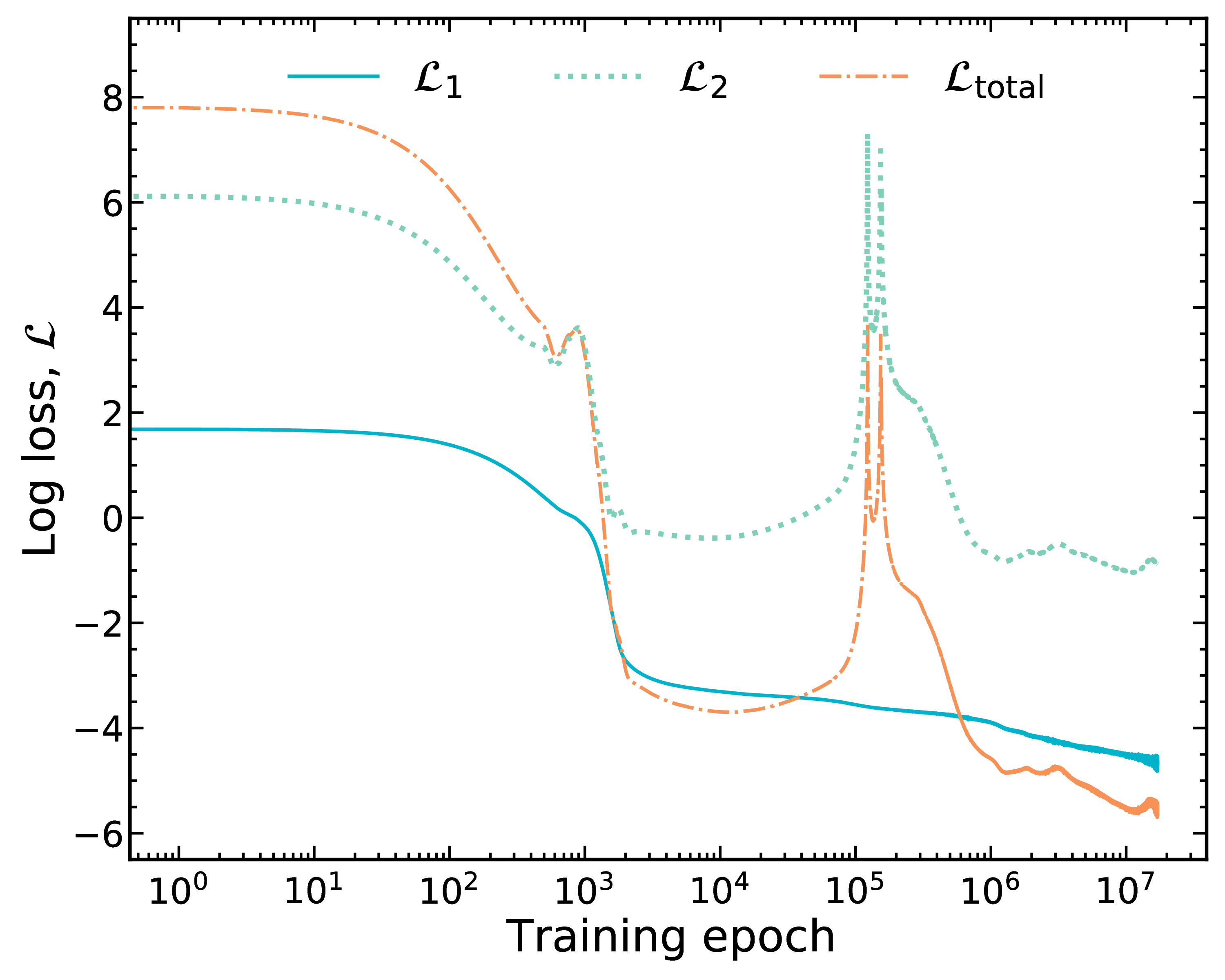$\mathcal{L}_\textrm{total} = \mathcal{L}_1 + \lambda_\textrm{phys} \mathcal{L}_2$ ; $\lambda_\textrm{phys}=1$ in this case

Good match to data does not imply good physics (although by happenstance it is in the end in this case)!

Mumpower, Sprouse, Lovell, & Mohan arXiv:2203.10594 (2022)

## Mass trends along isotopic chain (Nd, $Z=60$)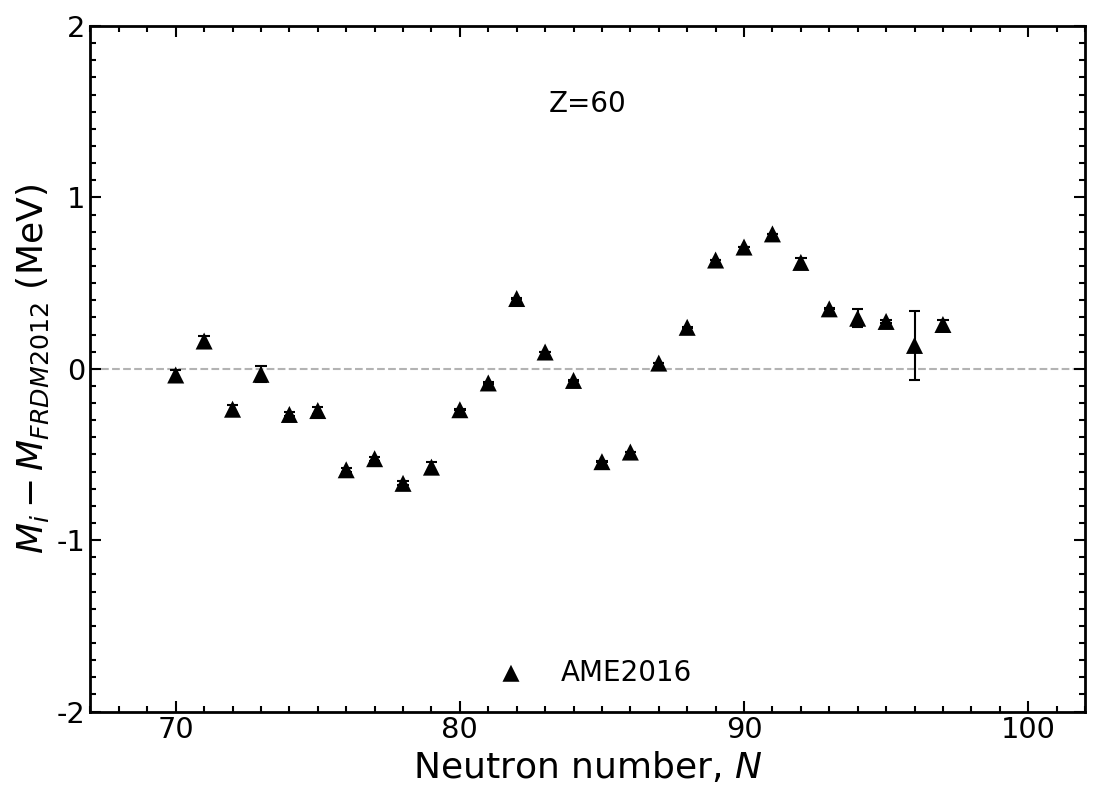Results plotted relative to the Los Alamos FRDM model

Modern mass models draw a 'straight line' through data

Mumpower, Sprouse, Lovell, & Mohan arXiv:2203.10594 (2022)

## Results of new model (Nd, $Z=60$)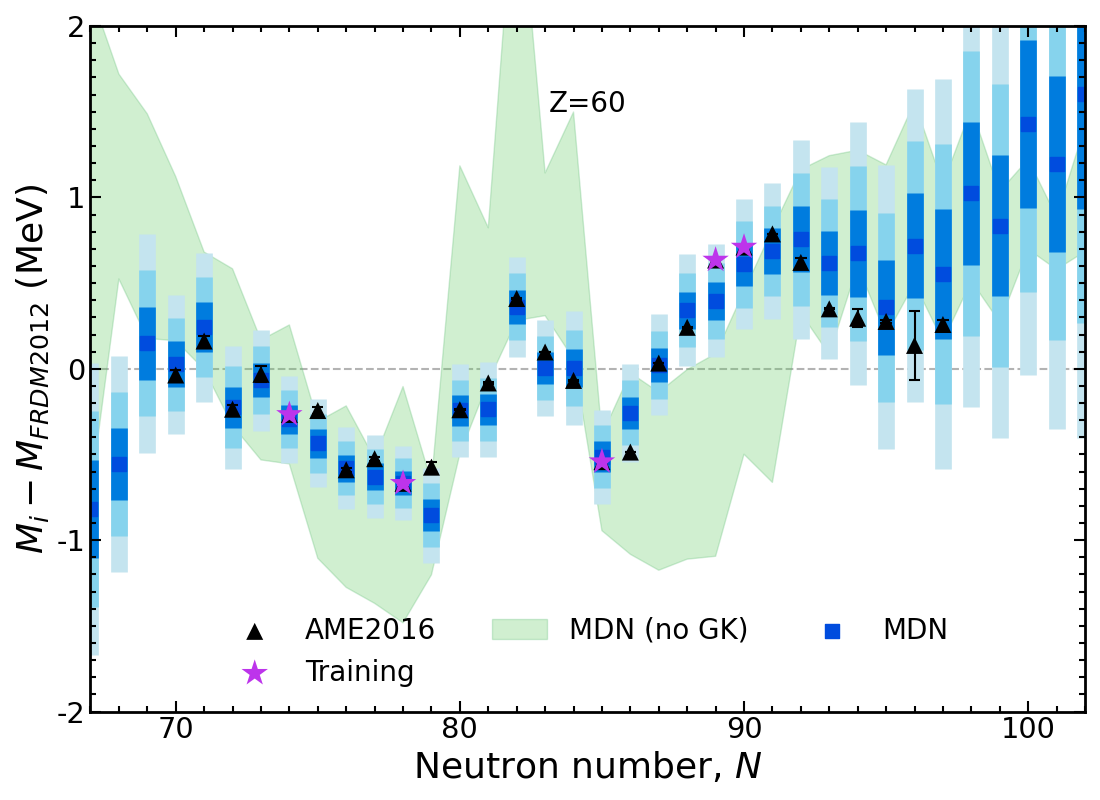Probabilistic network → well-defined uncertainties predicted for every nucleus ( 1$\sigma$, 2$\sigma$ 3$\sigma$)

Trends in data are captured far better than any mass model

Mumpower, Sprouse, Lovell, & Mohan arXiv:2203.10594 (2022)

## Results of new model (Nd, $Z=60$)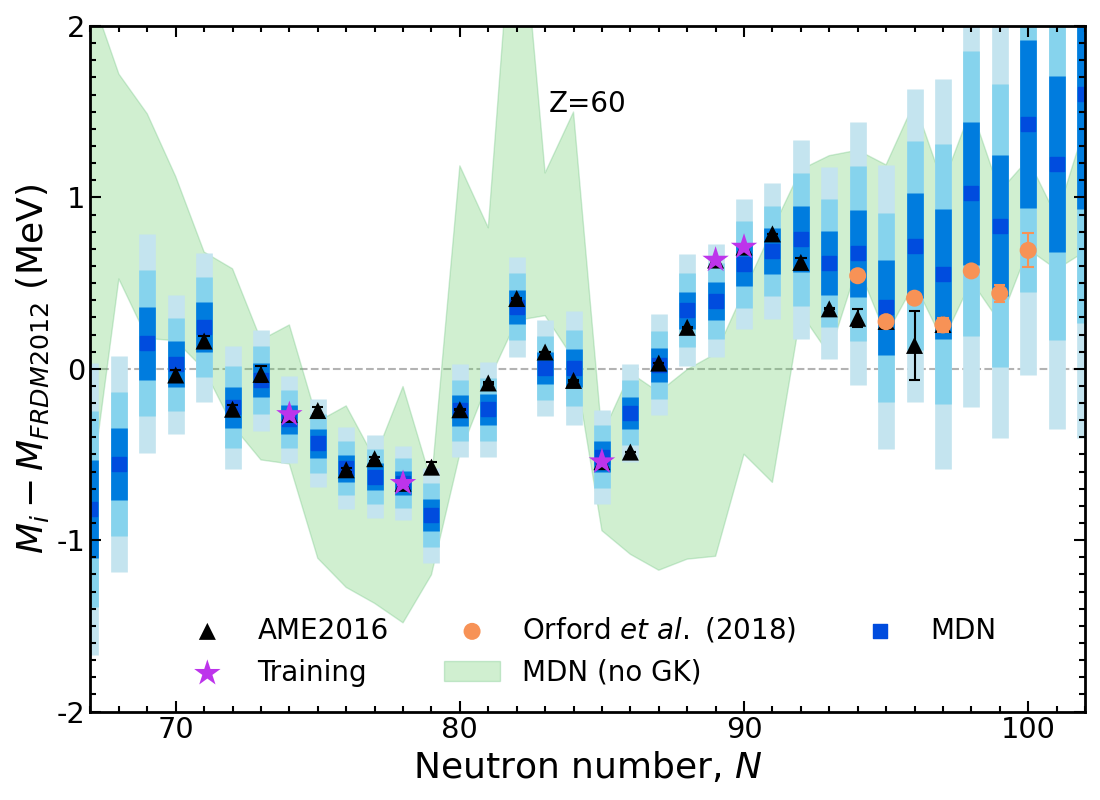New data (unknown to the model) are in agreement with the MDN model predictions

Model is now trained on only 20% of the AME and predicting 80%!

The training set is random; different datasets perform differently

Mumpower, Sprouse, Lovell, & Mohan arXiv:2203.10594 (2022)

## Results of new model (In, $Z=49$)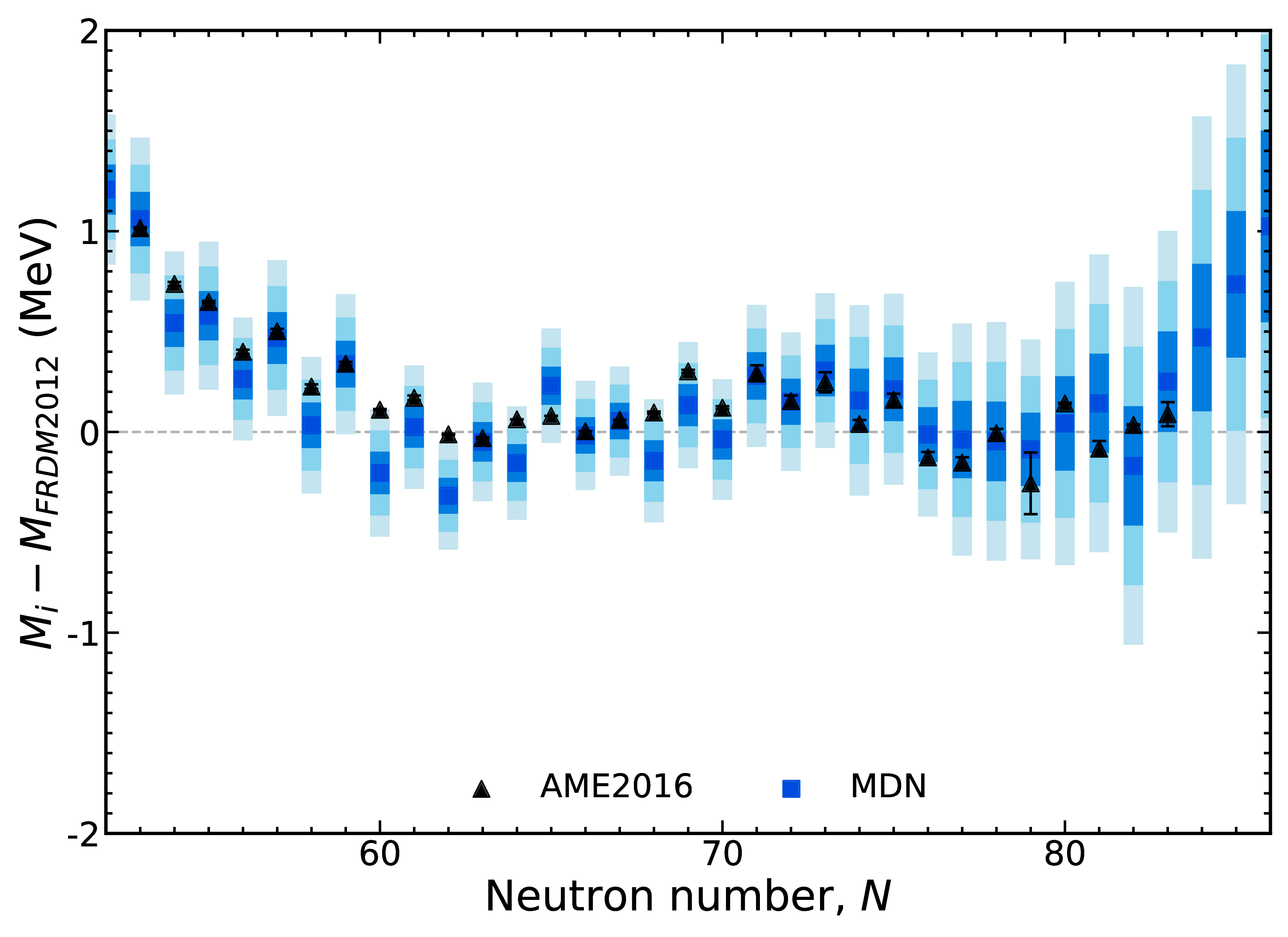Our results are not limited to any mass region ($Z \geq 20$) - all of them are described equally well

Results are better than any existing mass model; uncertainties inherently increase with extrapolation

We can predict new measurements and capture trends (important for astrophysics)

Mumpower, Sprouse, Lovell, & Mohan arXiv:2203.10594 (2022)

## How can we interpret these results?

One method is to calculate Shapley values (Lundberg & Lee 2017)

Idea arises in Game Theory when a coalition of players (ML - features) optimize gains relative to costs

The players may contribute unequally, but the payoff is mutually beneficial

Applicable to many applications, ML in particular to understand feature importance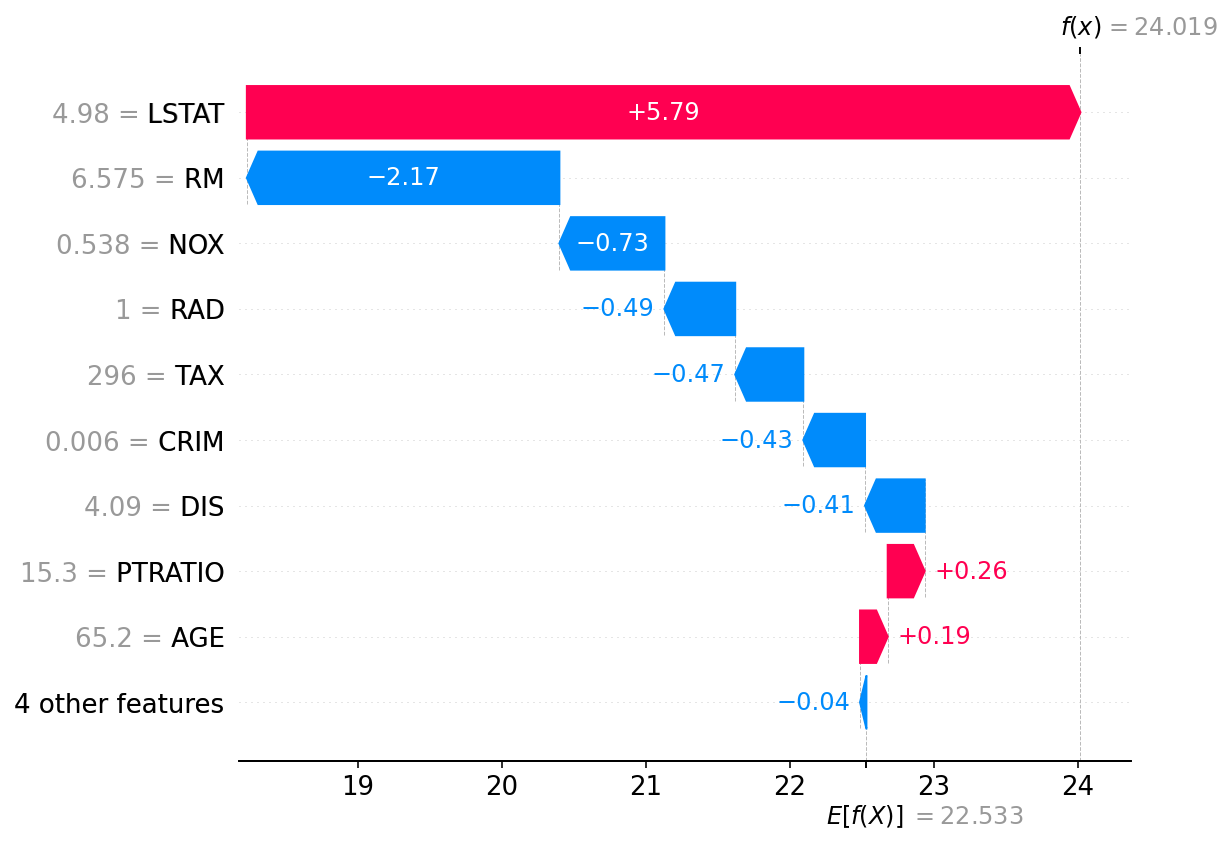Lipovetsky, Stan, and Michael Conklin, Applied Stochastic Models in Business and Industry (2001) • Strumbelj, Erik, and Kononenko, Knowledge and information systems (2014) • Figure from SHAP Python package (https://shap.readthedocs.io/)

## Ranking feature importance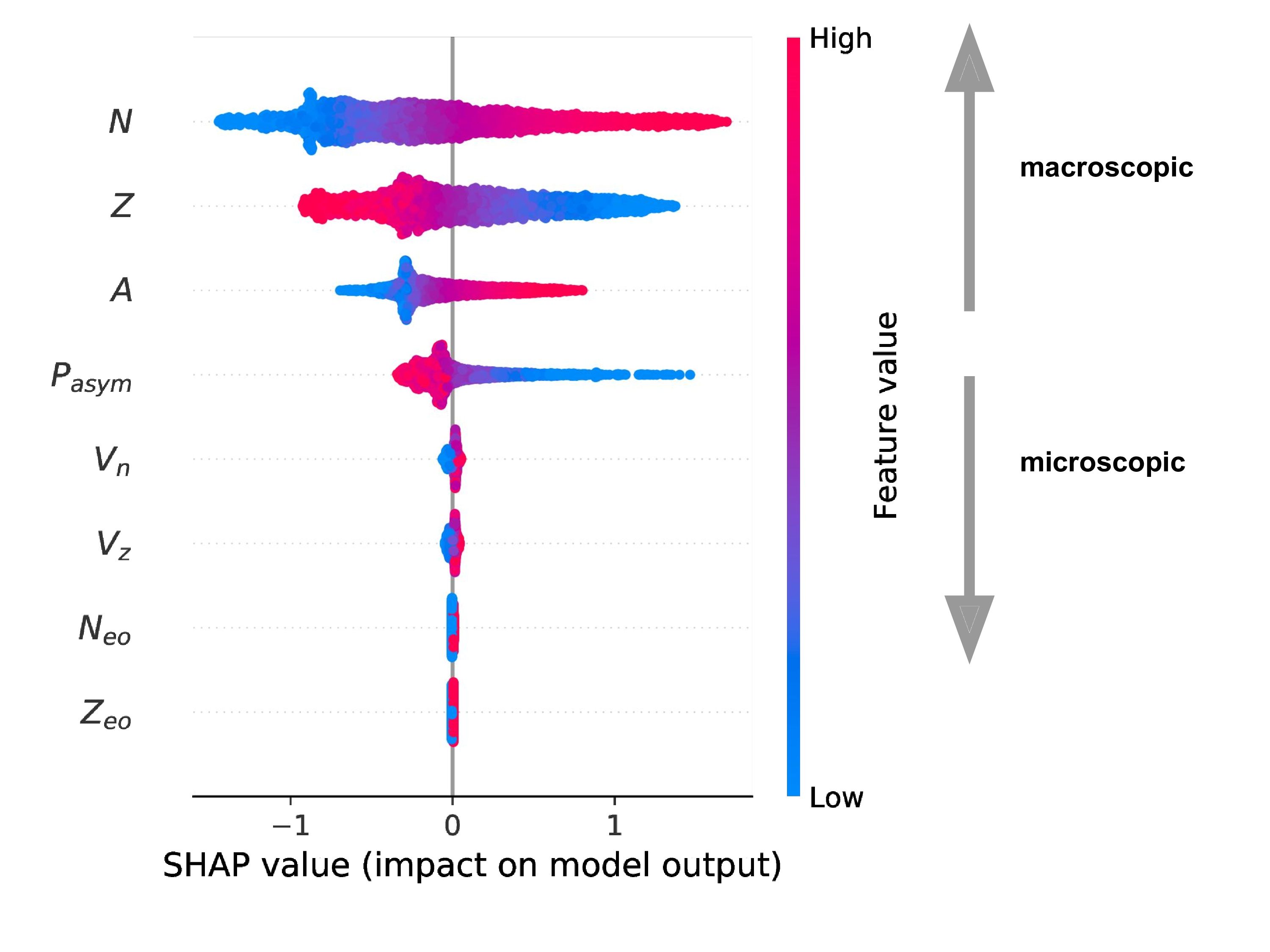SHAP calculated for all of the AME (2020) [predictions!]

The model behaves like a theoretical mass model

Macroscopic variables most important followed by quantum corrections

Mumpower, Sprouse, Lovell, & Mohan arXiv:2203.10594 (2022)

## Special thanks to

My collaborators

A. Lovell, A. Mohan, & T. Sprouse

PostdocAnd to my son Zachary for the use of his drawings

## Summary

We have developed an interpretable neural network capable of predicting masses

We can predict masses accurately and suss out trends in data

Our network is probabilistic, each prediction has an associated uncertainty

Our model can extrapolate and retains the physics

The quality of the training data is important (not shown)

We can classify the information content of existing and future measurements with this type of modeling

This modeling is general, and can be applied to any nuclear property with sufficient data

Results / Data / Papers @ MatthewMumpower.com